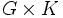# Corollary of Krull-Remak-Schmidt theorem for cancellation of direct factors

## Statement

Suppose$G,H,K$ are groups such that both$G$ and$H$ satisfy the ascending chain condition on normal subgroups and descending chain condition on normal subgroups. Then, the external direct product$G \times H$ is isomorphic to the external direct product$G \times K$, we must also have that$H$ and$K$ are isomorphic groups.

## Facts used

1. Krull-Remak-Schmidt theorem